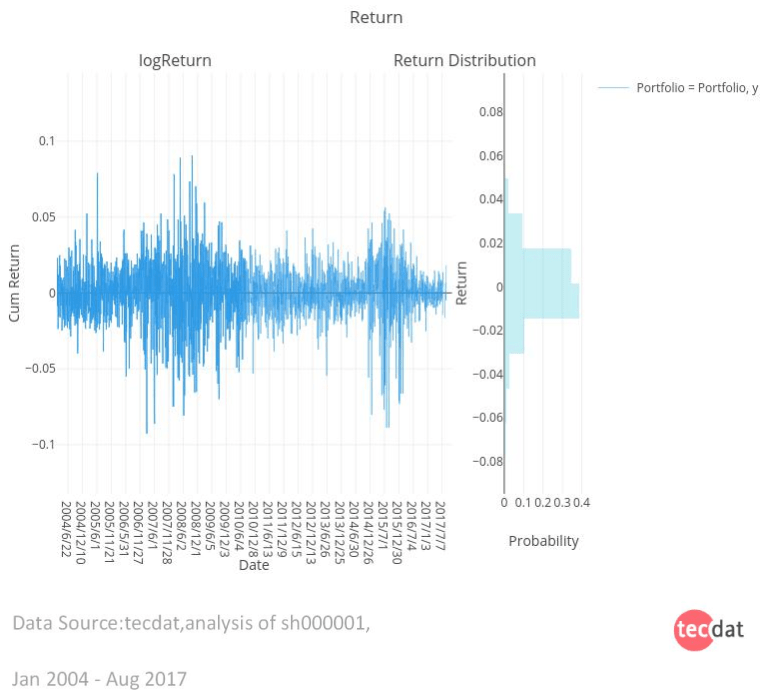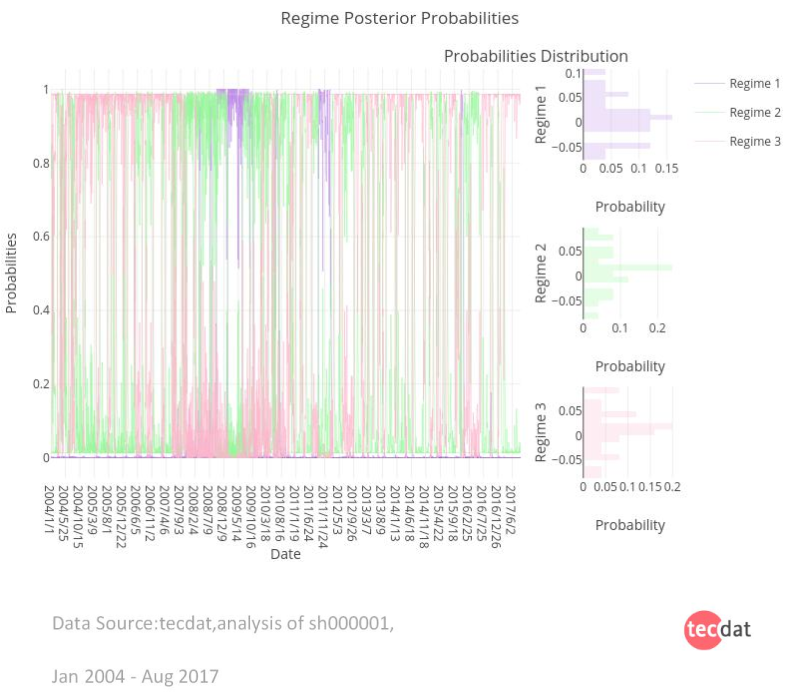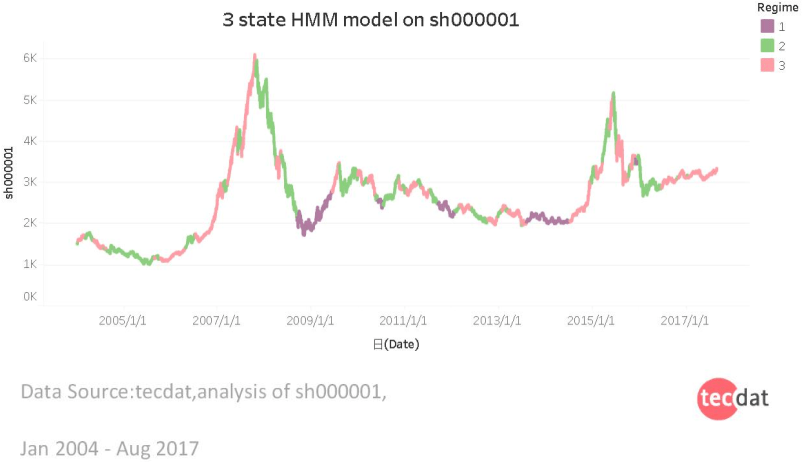# 构建模型

``````
df <- data.frame(Date=index(shdata),coredata(shdata))

# 创建布林带
bbands <- BBands(shdata[,c("000001.SS.High","000001.SS.Low","000001.SS.Close")])

# 连接和数据子集
df <- subset(cbind(df, data.frame(bbands[,1:3])), Date >= "2015-02-14")

#用于增长和减少的颜色列
for (i in 1:length(df[,1])) {
if (df\$X000001.SS.Close[i] >= df\$X000001.SS.Open[i]) {
df\$direction[i] = 'Increasing'
} else {
df\$direction[i] = 'Decreasing'
}
}

i <- list(line = list(color = '#17BECF'))
d <- list(line = list(color = '#7F7F7F'))

# 绘制烛台图

# 绘制条形图
pp <- df %>%
plot_ly(x=~Date, y=~X000001.SS.Volume, type='bar', name = "000001.SS Volume",
color = ~direction, colors = c('#17BECF','#7F7F7F')) %>%
layout(yaxis = list(title = "Volume"))

# 创建范围选择器按钮
rs <- list(visible = TRUE, x = 0.5, y = -0.055,
xanchor = 'center', yref = 'paper',
font = list(size = 9),
buttons = list(
...)
))

# 具有共享x轴的子图
p <- subplot(p, pp, heights = c(0.7,0.2), nrows=2,
shareX = TRUE, titleY = TRUE) %>%
layout(title = paste("000001.SS: 2004-01-01 -",Sys.Date()),
xaxis = list(rangeselector = rs),
legend = list(orientation = 'h', x = 0.5, y = 1,
xanchor = 'center', yref = 'paper',
font = list(size = 10),
bgcolor = 'transparent'))

p

``````2007 – 2009年间，由于次贷危机，股市出现了惊人的波动，迅速改变了不同状态的后验概率# R语言用隐马尔可夫模型HMM进行股票预测Kaizong Ye拓端研究室（TRL）的研究员。

​非常感谢您阅读本文，如需帮助请联系我们！QQ在线咨询

15121130882

0571-63341498

## 关注有关新文章的微信公众号

This will close in 0 seconds